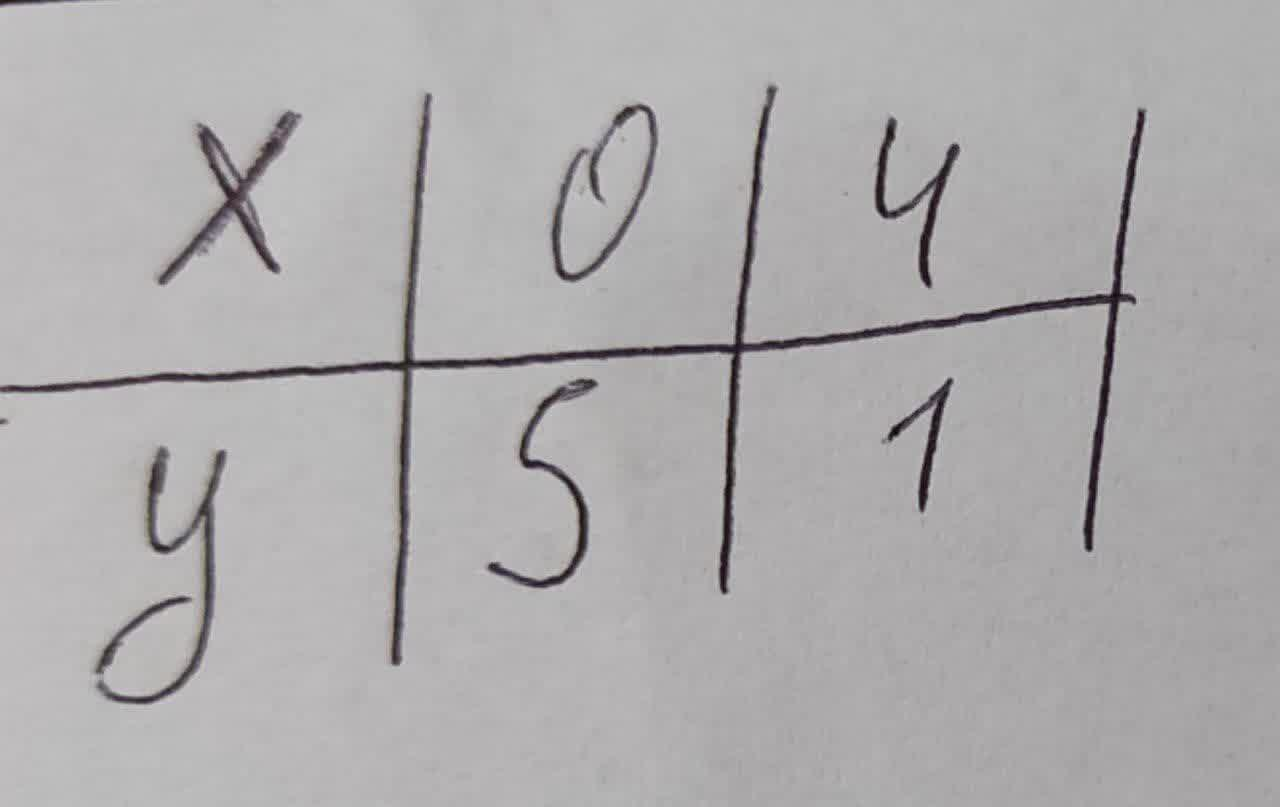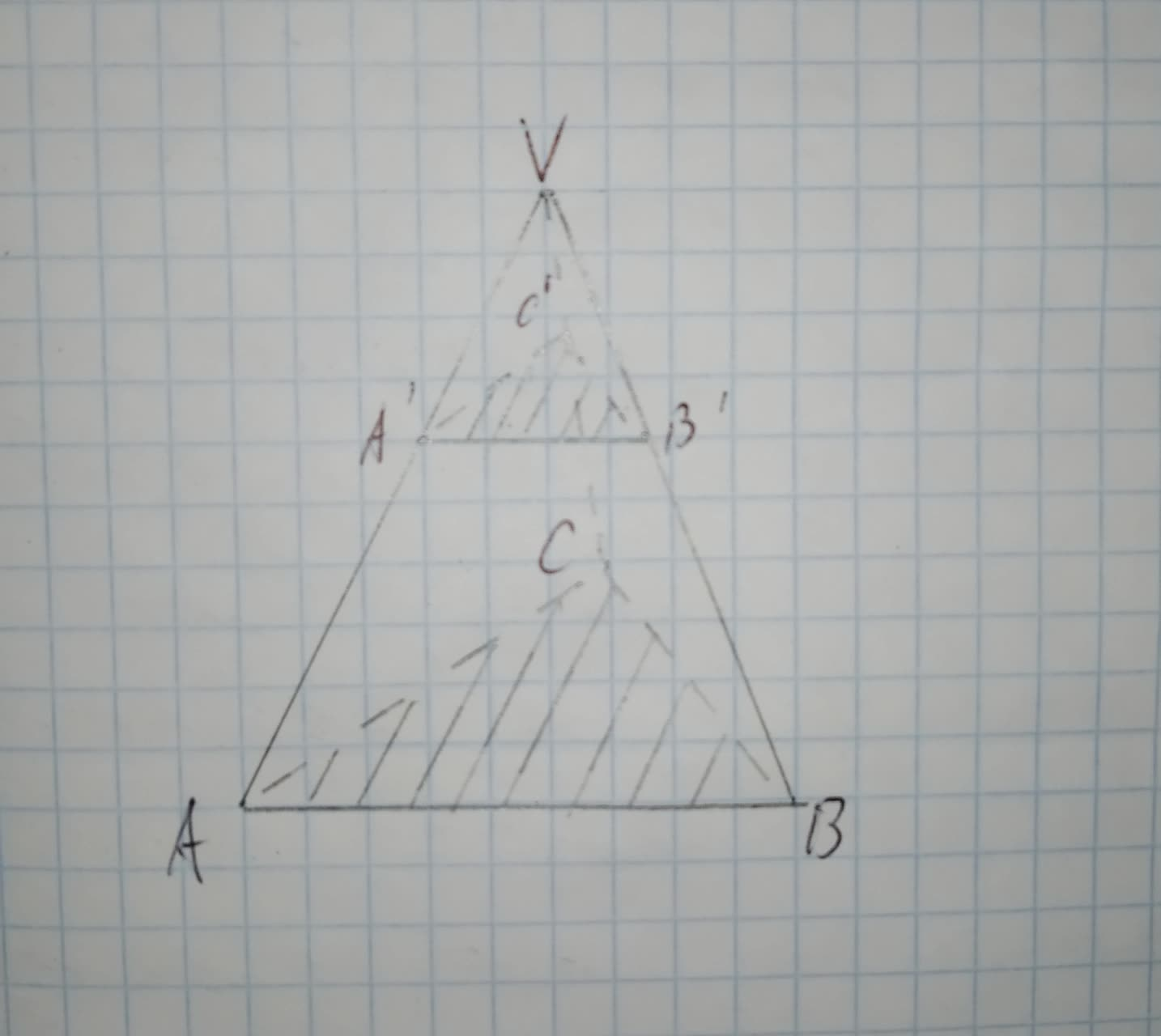# Find Expontntial Model that fits the points shown in the graph of table01510102841.jpgQuestion
Exponential modelsFind Expontntial Model that fits the points shown in the graph of table2020-12-18
Let us consider the following exponential model
$$\displaystyle{y}={a}{e}^{{{x}}}$$
Now, with given point (0,5), the equation becomes
$$\displaystyle{5}={a}{e}^{{{b}{\left({0}\right)}}}$$
$$\displaystyle{5}={a}{e}^{{0}}$$
Therefore,
a=5
With the given point (4,1) and a=1, the equation becomes
$$\displaystyle{1}={5}{e}^{{{b}{\left({4}\right)}}}$$
Dividing both sides by 5
$$\displaystyle\frac{{1}}{{5}}={e}^{{{4}{b}}}$$
Taking natural logarithm to the base e
$$\displaystyle\frac{{\ln{{1}}}}{{5}}={{\ln{{e}}}^{{{4}{b}}}}$$
Using the inverse property $$\displaystyle{{\ln{{e}}}^{{x}}}$$
$$\displaystyle\frac{{\ln{{1}}}}{{5}}={4}{b}$$
Therefore,
$$\displaystyle{b}=\frac{{\frac{{\ln{{1}}}}{{5}}}}{{4}}$$
b=-0.4024
Hence, the equation of the curve with a=5 and b=-0.4024 is $$\displaystyle{y}={e}^{{-{0.4024}{x}}}$$

### Relevant QuestionsFind the exponential model that fits the points shown in the graph. (Round the exponent to four decimal places)Often new technology spreads exponentially. Between 1995 and 2005, each year the number of Internet domain hosts was 1.43 times the number of hosts in the preceding year. In 1995, the number of hosts was 8.2 million.
(a) Explain why the number of hosts is an exponential function of time. The number of hosts grows by a factor of -----? each year, this is an exponential function because the number is growing by ------? decreasing constant increasing multiples.
(b) Find a formula for the exponential function that gives the number N of hosts, in millions, as a function of the time t in years since 1995.
(c) According to this model, in what year did the number of hosts reach 49 million?Use the exponential growth model, $$A=A_0e^{kt}$$. In 1975, the population of Europe was 679 million. By 2015, the population had grown to 746 million.
Solve,
a. Find an exponential growth function that models the data for 1975 through 2015.
b. By which year, to the nearest year, will the European population reach 800 million?For the following exercises, use a graphing utility to create a scatter diagram of the data given in the table. Observe the shape of the scatter diagram to determine whether the data is best described by an exponential, logarithmic, or logistic model. Then use the appropriate regression feature to find an equation that models the data. When necessary, round values to five decimal places.
$$\displaystyle{b}{e}{g}\in{\left\lbrace{a}{r}{r}{a}{y}\right\rbrace}{\left\lbrace{\left|{c}\right|}{c}{\mid}\right\rbrace}{h}{l}\in{e}{x}&{1}&{2}&{3}&{4}&{5}&{6}&{7}&{8}&{9}&{10}\backslash{h}{l}\in{e}{f{{\left({x}\right)}}}&{409.4}&{260.7}&{170.4}&{110.6}&{74}&{44.7}&{32.4}&{19.5}&{12.7}&{8.1}\backslash{h}{l}\in{e}{e}{n}{d}{\left\lbrace{a}{r}{r}{a}{y}\right\rbrace}$$To determine:To Find:the measure of side BB' in the figure shown such that VA' = 15, A'A=20 and A'B'=24
Given:
Figure is shown below.To determine:To Find:the measure of side BB' in the figure shown such that VA' = 15, A'A=20 and VB=49
Given:
Figure is shown below.To determine:To Find:the measure of side VC in the figure shown such that VA' = 15, A'A=20 and VC'=18
Given:
Figure is shown below.The annual sales S (in millions of dollars) for the Perrigo Company from 2004 through 2010 are shown in the table. $$\displaystyle{b}{e}{g}\in{\left\lbrace{a}{r}{r}{a}{y}\right\rbrace}{\left\lbrace{\left|{c}\right|}{c}{\mid}\right\rbrace}{h}{l}\in{e}\text{Year}&{2004}&{2005}&{2006}&{2007}&{2008}&{2009}&{2010}\backslash{h}{l}\in{e}\text{Sales, S}&{898.2}&{1024.1}&{1366.8}&{1447.4}&{1822.1}&{2006.9}&{2268.9}\backslash{h}{l}\in{e}{e}{n}{d}{\left\lbrace{a}{r}{r}{a}{y}\right\rbrace}$$ a) Use a graphing utility to create a scatter plot of the data. Let t represent the year, with $$\displaystyle{t}={4}$$ corresponding to 2004. b) Use the regression feature of the graphing utility to find an exponential model for the data. Use the Inverse Property $$\displaystyle{b}={e}^{{{\ln{\ }}{b}}}$$ to rewrite the model as an exponential model in base e. c) Use the regression feature of the graphing utility to find a logarithmic model for the data. d) Use the exponential model in base e and the logarithmic model to predict sales in 2011. It is projected that sales in 2011 will be \$2740 million. Do the predictions from the two models agree with this projection? Explain.Use the exponential growth model $$A=A_0e^{kt}$$ to solve: In 2000, there were 110 million cellphone subscribers in the United States. By 2010, there were 303 million subscribersUse the exponential growth model $$\displaystyle{A}={A}_{{0}}{e}^{{{k}{t}}}$$ to solve: In 2000, there were 110 million cellphone subscribers in the United States. By 2010, there were 303 million subscribers